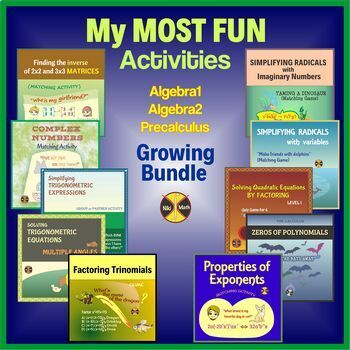# Algebra 1,2 & Precalculus Curriculum:MY MOST FUN Activities(DIGITAL + Google Sl)Subject
Resource Type
Format
Zip (158 MB|N/A)TpT Digital ActivityGoogle Slides™
Standards
\$304.24
Bundle
List Price:
\$470.95
Bundle Price:
\$320.25
You Save:
\$166.71
\$304.24
Bundle
List Price:
\$470.95
Bundle Price:
\$320.25
You Save:
\$166.71This bundle contains one or more resources with Google apps (eg. docs, slides, etc.).TpT Digital Activity Included
This bundle contains one or more resources that include an interactive version that students can complete from any device on TpT’s new tool. Learn more.

#### Products in this Bundle (150)

showing 1-5 of 150 products

### Description

(THE ITEMS INCLUDED HERE ARE ALL TURNED INTO DIGITAL!There are included online resources (Google Slide products) as well.)

These are my MOST FUN Algebra 1, 2 and PreCalculus activities (partner and group activities, independent activities, task cards, matching games, cut and paste, maze, answer pass, complete the story, card sort, drawing activities, unique new activities like password search activity, make compound words activity, find out the idioms, hidden constellation, find the names of 12 stars/asteroids, etc ) . The products are designed to strengthen and reinforce student learning and make students enjoy while solving the problems.

The packet represents 32% savings off of the items if purchased individually.

The resource covers the following topics:

ALGEBRA 1 & 2

◈ Order of Operations

◈ Distributive Property

◈ Combining Like Terms

◈ Simplifying and Evaluating Algebraic Expressions

◈ Scientific Notation

◈ Solving Proportions

◈ Adding , Subtracting, Multiplying and Dividing Polynomials (No Remainders)

◈ One Step Equations

◈ Multi - Step Equations and Inequalities

◈ Absolute Value Equations and Inequalities

◈ Systems of Linear Inequalities

◈ Factoring Polynomials by Determining a GCF

◈ Factoring Difference of Squares

◈ Factoring by Grouping

◈ Factoring Trinomials

◈ Simplifying Radical Expressions - Rationalizing the Denominator

◈ Simplifying Radical Expressions with Imaginary Numbers

◈ Properties of Exponents - Simplifying Exponential Expressions

◈ Operations with Complex Numbers

◈ Distance Between Two Points

◈ Slope Between Two Points

◈ Writing a Linear Equation

◈ Solving Systems of Linear Equations

- algebraically ( substitution and elimination methods)

- graphically

◈ Solving Quadratic Equations with Real ( Rational and Irrational) Solutions in Standard, Vertex and Other forms

- by the square root method

- by factoring

- by all methods

◈ Quadratic Equations with Complex Solutions

◈ Pascal's Triangle and Binomial Theorem (Expanding Binomials)

◈ Completing the Square

◈ Quadratic Functions - Converting, Analyzing and Graphing

◈ Simplifying Rational Expressions

◈ Operations with Rational Expressions

◈ Rational Equations Reducible to Linear Equations ( solving by cross multiplying)

◈ Solving Rational Equations Reducible to Quadratic Equations

- by cross multiplying

- by multiplying by the LCD

- by factoring to find the LCD

◈ Rational Equations with Extraneous Solutions

◈ Radical Equations with No Solution

◈ Graphing Exponential Functions

PRECALCULUS

◈ Domain of Functions

◈ Composition of Functions

◈ Operation on Functions

◈ Inverse Functions

◈ Even and Odd Functions

◈ Zeros of Polynomials - Real & Complex

◈ Graphing Polynomials

◈ Partial Fractions Decomposition

◈ Dividing Polynomials Using Long Division

◈ Solving Exponential Equations without Using Logarithms

◈ Evaluating Logarithms and Logarithmic Expressions

◈ Expanding and Condensing Logarithms

◈ Change of Base Formula

◈ Solving Exponential Equations Using Logarithms

◈ Solving Logarithmic Equations

◈ Solving Rational Inequalities

◈ Law of Sines

◈ Law of Cosines

◈ Area of Oblique Triangles

◈ Converting between Radians and Degrees

◈ Converting between Polar and Rectangular Coordinates

◈ Arc Length and Sector Area

◈ Simplifying Trigonometric Expressions by using fundamental trigonometric identities (Pythagorean, Quotient, Reciprocal, Co-function Identities)

◈ Proving and Disproving Trigonometric Identities

◈ Solving Trigonometric Equations

• by Factoring • by the Quadratic Formula • by All Methods

• by using the fundamental trigonometric identities

◈ Finding Determinants of 2x2 and 3x3 Matrices

◈ Adding, Subtracting and Scalar Multiplication of Matrices

◈ Multiplying Matrices

◈ Inverse of 2x2 and 3x3 Matrices

◈ Solving Matrix Equations

◈ Solving Linear Systems with Three Variables

◈ Dot Product of Two Vectors

******************************

You might be also interested in

1) My ALL Algebra 1 & 2 products included in ALGEBRA 1 & 2 Big Growing Activities Bundle - 32% SAVINGS

1) My ALL Precalculus products included in BIG Growing PRECALCULUS Activities Bundle - 32% SAVINGS

4) My ALL Calculus products included in AP Calculus 1&2 (AB & BC) Big Growing Activities Bundle - 32% OFF

Total Pages
N/A
Included
Teaching Duration
N/A
Report this Resource to TpT
Reported resources will be reviewed by our team. Report this resource to let us know if this resource violates TpT’s content guidelines.

### Standards

to see state-specific standards (only available in the US).
Prove the addition and subtraction formulas for sine, cosine, and tangent and use them to solve problems.
Use inverse functions to solve trigonometric equations that arise in modeling contexts; evaluate the solutions using technology, and interpret them in terms of the context.
Understand radian measure of an angle as the length of the arc on the unit circle subtended by the angle.
Understand the inverse relationship between exponents and logarithms and use this relationship to solve problems involving logarithms and exponents.
Verify by composition that one function is the inverse of another.